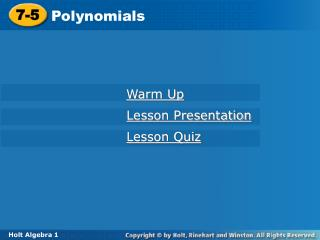# 7-5 - PowerPoint PPT PresentationDownload Presentation7-5

7-5Download Presentation## 7-5

- - - - - - - - - - - - - - - - - - - - - - - - - - - E N D - - - - - - - - - - - - - - - - - - - - - - - - - - -
##### Presentation Transcript

1. 7-5 Polynomials Warm Up Lesson Presentation Lesson Quiz Holt Algebra 1

2. Warm Up Evaluate each expression for the given value of x. 1. 2x + 3; x = 22.x2+ 4; x = –3 3. –4x – 2; x = –1 4. 7x2 + 2x = 3 Identify the coefficient in each term. 5. 4x36. y3 7. 2n78. –54 7 13 2 69 4 1 –1 2

3. Objectives Classify polynomials and write polynomials in standard form. Evaluate polynomial expressions.

4. Vocabulary monomial degree of a monomial polynomial degree of a polynomial standard form of a polynomial leading coefficient quadratic cubic binomial trinomial

5. A monomial is a number, a variable, or a product of numbers and variables with whole-number exponents. The degree of a monomial is the sum of the exponents of the variables. A constant has degree 0.

6. A. 4p4q3 Example 1: Finding the Degree of a Monomial Find the degree of each monomial. The degree is 7. Add the exponents of the variables: 4 + 3 = 7. B. 7ed C. 3

7. a. b. b. 1.5k2m 4x 2c3 Check It Out! Example 1 Find the degree of each monomial.

8. A polynomialis a monomial or a sum or difference of monomials. The degree of a polynomial is the degree of the term with the greatest degree.

9. B. Example 2: Finding the Degree of a Polynomial Find the degree of each polynomial. A. 11x7 + 3x3 11x7: degree 7 3x3: degree 3 Find the degree of each term. The degree of the polynomial is the greatest degree, 7.

10. Check It Out! Example 2 Find the degree of each polynomial. a. 5x – 6 b. x3y2 + x2y3 – x4 + 2

11. The terms of a polynomial may be written in any order. However, polynomials that contain only one variable are usually written in standard form. The standard form of a polynomial that contains one variable is written with the terms in order from greatest degree to least degree. When written in standard form, the coefficient of the first term is called the leading coefficient.

12. 6x – 7x5 + 4x2 + 9 –7x5 + 4x2 + 6x + 9 2 Degree 1 5 2 5 1 0 0 –7x5 + 4x2 + 6x + 9. The leading The standard form is coefficient is –7. Example 3A: Writing Polynomials in Standard Form Write the polynomial in standard form. Then give the leading coefficient. 6x – 7x5 + 4x2 + 9 Find the degree of each term. Then arrange them in descending order:

13. Example 3B: Writing Polynomials in Standard Form Write the polynomial in standard form. Then give the leading coefficient. y2 + y6 − 3y

14. Check It Out! Example 3a Write the polynomial in standard form. Then give the leading coefficient. 16 – 4x2 + x5 + 9x3

15. Check It Out! Example 3b Write the polynomial in standard form. Then give the leading coefficient. 18y5 – 3y8 + 14y

16. Terms Name 1 Monomial 0 Constant 2 Binomial 1 Linear 3 Trinomial Quadratic 2 Polynomial 4 or more Cubic 3 Quartic 4 Quintic 5 6 or more 6th,7th,degree and so on Some polynomials have special names based on their degree and the number of terms they have.

17. Example 4: Classifying Polynomials Classify each polynomial according to its degree and number of terms. A. 5n3 + 4n 5n3 + 4n is acubic binomial. Degree 3 Terms 2 B. 4y6 – 5y3 + 2y – 9 C. –2x

18. Classify each polynomial according to its degree and number of terms. D. x3 + x2 – x + 2 E. 6 F. –3y8 + 18y5+ 14y

19. Example 5: Application A tourist accidentally drops her lip balm off the Golden Gate Bridge. The bridge is 220 feet from the water of the bay. The height of the lip balm is given by the polynomial –16t2 + 220, where t is time in seconds. How far above the water will the lip balm be after 3 seconds?

20. Lesson Quiz: Part I Find the degree of each polynomial. 1. 7a3b2 – 2a4 + 4b –15 2. 25x2 – 3x4 Write each polynomial in standard form. Then give the leading coefficient. 3. 24g3 + 10 + 7g5 – g2 4. 14 – x4 + 3x2 5 4 7g5 + 24g3 – g2 + 10; 7 –x4 + 3x2 + 14; –1

21. Lesson Quiz: Part II Classify each polynomial according to its degree and number of terms. quadratic trinomial 5. 18x2 – 12x + 5 6. 2x4 – 1 quartic binomial 7. The polynomial 3.675v + 0.096v2 is used to estimate the stopping distance in feet for a car whose speed is y miles per hour on flat dry pavement. What is the stopping distance for a car traveling at 70 miles per hour? 727.65 ft